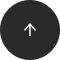Standard SQL

# How to Handle Divide by Zero In SQL

NULLIF, WHERE

#### Problem:

You want to perform division in your SQL query, but the denominator is an expression that can be zero. The database will give you an error when the denominator is in fact zero.

#### Example:

Our database has a table named `investor_data` with data in the following columns: `id`, `investor_year`, `price_per_share`, `income`, and `expenses`.

idinvestor_yearprice_per_shareincomeexpenses
120162032002300
2201713020002000
3201840200100
420191559004900

Let’s divide the price per share by the difference between income and expenses to determine the P/E ratio (price-earnings ratio) for each year. Notice that there are cases when income equals expenses, so their difference will be zero. Thus, we need to find a way to avoid division by zero.

#### Solution:

```SELECT investor_year,
price_per_share/NULLIF(income-expenses, 0)  AS P_E_ratio
FROM investor data;
```

This query returns the P/E ratio for each year as described in the problem statement:

investor_yearP_E_ratio
20160.0222
2017NULL
20180.4000
20190.0150

If the difference between income and expenses is zero (as is the case for 2017), the `NULLIF` function changes the zero to a NULL value. Therefore, division by zero gives you NULL in the result.

#### Discussion:

If you’d like to handle division by zero gracefully, you can use the `NULLIF` function. `NULLIF` takes two arguments: the expression of interest, and the value you want to override. If the first argument is equal to the second, then `NULLIF` returns NULL; otherwise, it returns the first argument.

You can use this function to handle a potential division by zero by wrapping the denominator in a call to `NULLIF`. In our example if the difference between income and expenses is zero, this value will be changed to NULL, and the denominator in the division will be NULL, not zero.

#### Solution 2: Use `WHERE`

Of course, in some situations, you can use a simpler solution: Just avoid division by zero by using `WHERE` with the comparison operator <>. In our example, we could check if income-expenses is different than 0. If it is, the calculation will be returned.

```SELECT investor_year,
price_per_share/(income-expenses) AS P_E_ratio
FROM investor data
WHERE (income-expenses) <> 0 ;
```

Note that with the `WHERE` solution, you’ll get fewer rows than there are in the `investor_date` table. The rows where income and expenses are equal will not be shown in the final result.

#### Recommended courses: